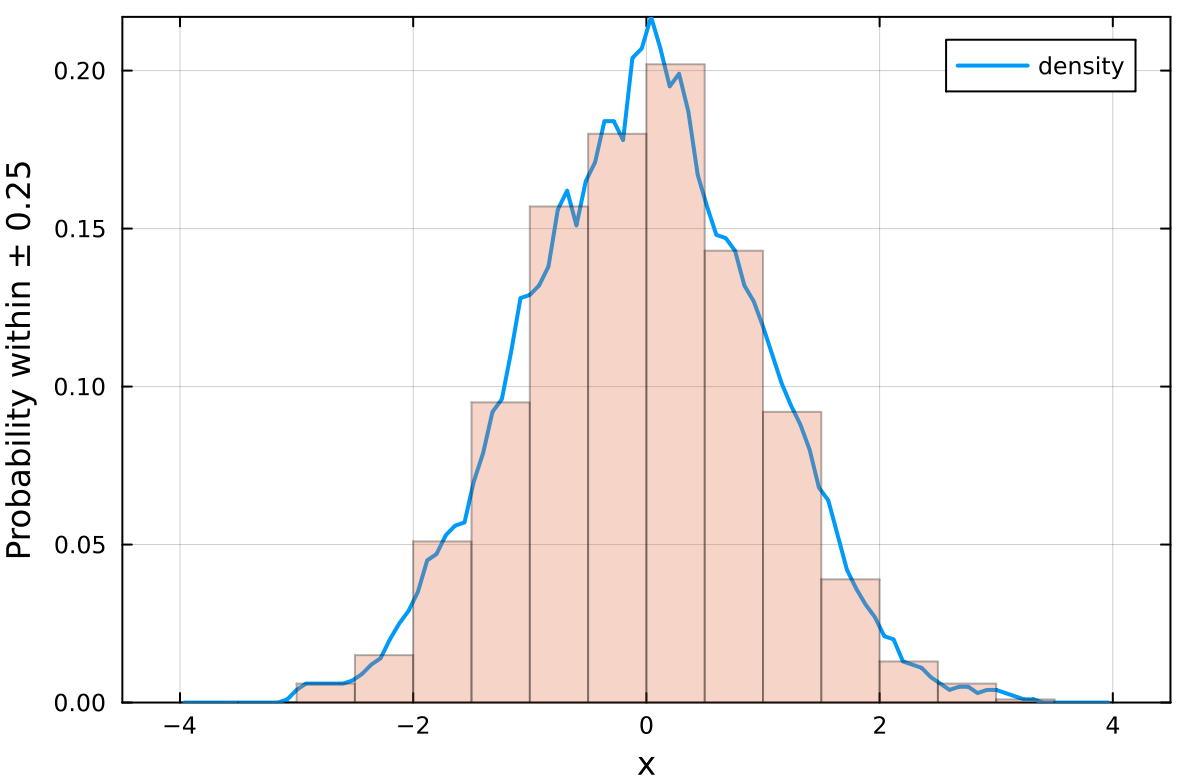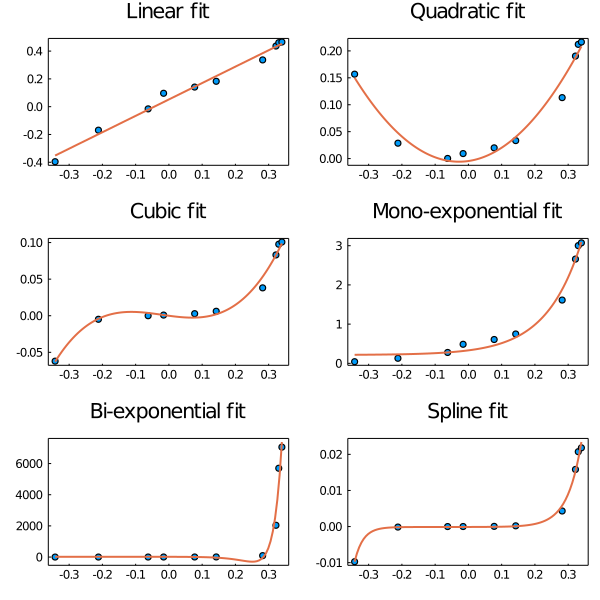## EasyFit.jl

Easy interface for obtaining fits for 2D data
Author m3g
Popularity
5 Stars
Updated Last
2 Years Ago
Started In
August 2020

# EasyFit

Easy interface for obtaining fits of 2D data.

The purpose of this package is to provide a very simple interface to obtain some of the most common fits of 2D data. Currently, simple fitting functions are available for linear, quadratic, cubic, exponential, and spline fits.

On the background this interface uses the LsqFit and Interpolations, which are already quite easy to use. Additionally, EasyFit contains a simple globalization heuristic, such that good non-linear fits are obtained often.

Our aim is to provide a package for quick fits without having to think about the code.

## Installation

```julia> ] add EasyFit

julia> using EasyFit
```

## Contents

Read the `Linear fit` section first, because all the others are similar, with few specificities:

## Linear fit

To perform a linear fitting, do:

```julia> x = sort(rand(10)); y = sort(rand(10)); # some data

julia> fit = fitlinear(x,y)

------------------- Linear Fit -------------

Equation: y = ax + b

With: a = 0.9245529646308137
b = 0.08608398402393584

Pearson correlation coefficient, R = 0.765338307304594

Predicted y = [-0.009488291459872424, -0.004421217036880542...
Residues = [-0.08666886144188027, -0.12771486789744962...

--------------------------------------------

```

The `fit` data structure which comes out of `fitlinear` contains the output data with the same names as shown in the above output:

```julia> fit.a
0.9245529646308137

julia> fit.b
0.08608398402393584

julia> fit.R
0.765338307304594

```

The `fit.x` and `fit.y` vectors can be used for plotting the results:

```julia> using Plots

julia> scatter(x,y) # the original data

julia> plot!(fit.x,fit.y) # the fit

```

Use the `fitquad` function:

```julia> fitquad(x,y)  # or fitquadratic(x,y)

Equation: y = ax^2 + bx + c

With: a = 0.935408728589832
b = 0.07985866671623199
c = 0.08681962205579699

Pearson correlation coefficient, R = 0.765338307304594

Predicted y = [0.08910633345247763, 0.08943732276526263...
Residues = [0.07660191693062993, 0.07143385689027287...

-----------------------------------------------
```

## Cubic fit

Use the `fitcubic` function:

```julia> fitcubic(x,y)

------------------- Cubic Fit -----------------

Equation: y = ax^3 + bx^2 + cx + d

With: a = 1.6860182468269271
b = -2.197790204605215
c = 1.431666717127516
d = -0.10389199522825227

Pearson correlation coefficient, R = 0.765338307304594

Predicted Y: ypred = [0.024757602237563042, 0.1762724543346461...
residues = [-0.021614675301217884, 0.0668157091306878...

-----------------------------------------------

```

## Exponential fits

Use the `fitexp` function:

```julia> fitexp(x,y) # or fitexponential

------------ Single Exponential fit -----------

Equation: y = A exp(x/b) + C

With: A = 0.08309782657193134
b = 0.4408664103095801
C = 1.4408664103095801

Pearson correlation coefficient, R = 0.765338307304594

Predicted Y: ypred = [0.10558554154948542, 0.16605481935145136...
residues = [0.059213264010704494, 0.056598074147493044...

-----------------------------------------------

```

or add `n=N` for a multiple-exponential fit:

```julia> fit = fitexp(x,y,n=3)

-------- Multiple-exponential fit -------------

Equation: y = sum(A[i] exp(x/b[i]) for i in 1:3) + C

With: A = [2.0447736471832363e-16, 3.165225832379937, -3.2171314371600785]
b = [0.02763465220057311, -46969.25088088338, -4.403370258345724]
C = 3.543252432454542

Pearson correlation coefficient, R = 0.765338307304594

Predicted Y: ypred = [0.024313571992034433, 0.1635108558614995...
residues = [-0.022058705546746493, 0.05405411065754118...

-----------------------------------------------
```

## Splines

Use the `fitspline` function:

```julia> fit = fitspline(x,y)

-------- Spline fit ---------------------------

x spline: x = [0.10558878272489601, 0.1305310750202113...
y spline: y = [0.046372277538780926, 0.05201906296544364...

-----------------------------------------------
```

Use `plot(fit.x,fit.y)` to plot the spline.

## Moving Averages

Use the `movavg` (or `movingaverage`) function:

```julia> ma = movavg(x,y,50)

------------------- Moving Average ----------

Number of points averaged: 51 (± 25 points)

Pearson correlation coefficient, R = 0.9916417123050962

Averaged Y: y = [0.14243985510210114, 0.14809841636897675...
residues = [-0.14230394758154755, -0.12866864179092025...

--------------------------------------------

```

Use `plot(ma.x,ma.y)` to plot the moving average.

## Density function

Use the `fitdensity` to obtain the density function (continuous histogram) of a data set `x`:

```julia> x = randn(1000)

julia> density = fitdensity(x)

------------------- Density -------------

d contains the probability of finding data points within x ± 0.25

-----------------------------------------
```

Options are the step size (`step=0.5`) and normalization type (probability by default, with `norm=1` or number of data points, with `norm=0`).

Example:

``````julia> x = randn(1000);

julia> density = fitdensity(x,step=0.5,norm=0);

julia> histogram(x,xlabel="x",ylabel="Density",label="",alpha=0.3,framestyle=:box);

julia> plot!(density.x,density.d,linewidth=2);

``````## Bounds

Lower and upper bounds can be set to the parameters of each function using the `l=lower()` and `u=upper()` input parameters. For example:

```julia> fit = fitlinear(x,y,l=lower(a=5.),u=upper(a=10.))
```
```julia> fit = fitexp(x,y,n=2,l=lower(a=[0.,0]),u=upper(a=[1.,1.]))
```

Bounds to the intercepts or limiting values are not supported, but it is possible to set them to a constant value. For example:

```julia> fit = fitlinear(x,y,b=5.)
```
```julia> fit = fitexp(x,y,n=2,c=0.)
```

## Example output:

This figure was obtained using `Plots`, after obtaining a fit of each type, with

```julia> scatter!(x,y) # plot original data
julia> plot!(fit.x,fit.y) # plot the resulting fit
```## Options

It is possible to pass an optional set of parameters to the functions. Use, for example:

```julia> fitexp(x,y,options=Options(maxtrials=1000))
```

Available options:

Keyword Type Default value Meaning
`fine` `Int` 100 Number of points of fit to smooth plot.
`p0_range` `Vector{Float64,2}` `[-100*(maximum(Y)-minimum(Y)), 100*(maximum(Y)-minimum(Y))]` Range of gereneration of initial random parameters.
`nbest` `Int` 5 Number of repetitions of best solution in global search.
`besttol` `Float64` 1e-4 Similarity of the sum of residues of two solutions such that they are considered the same.
`maxtrials` `Int` 100 Maximum number of trials in global search.
`debug` `Bool` false Prints errors of failed fits.

### Used By Packages

No packages found.